If you have a related question, please click the "Ask a related question" button in the top right corner. The newly created question will be automatically linked to this question.

# OPA2210: TINA for OPA2210 stability

Part Number: OPA2210
Other Parts Discussed in Thread: OPA210

Hi,

I need to simulate the stability for OPA2210. I download the TINA model from TI website. And I have done a TINA simulation. But I am not sure CF and RISO value is correct.

OPA2210 input common capacitance is 0.5pF, and the input differential  capacitance is 9pF. so the input total capacitance is 9.5pF.

RF=1K, RI=5K.

Could you help me to check the TINA simulation and send me a correct OPA2210 TINA simulation for the stability?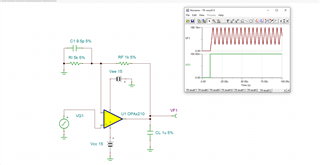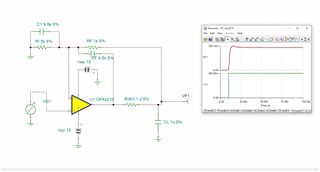OPA2210_4.5nF.TSC

• Hi Tengfei,But you could do it this way: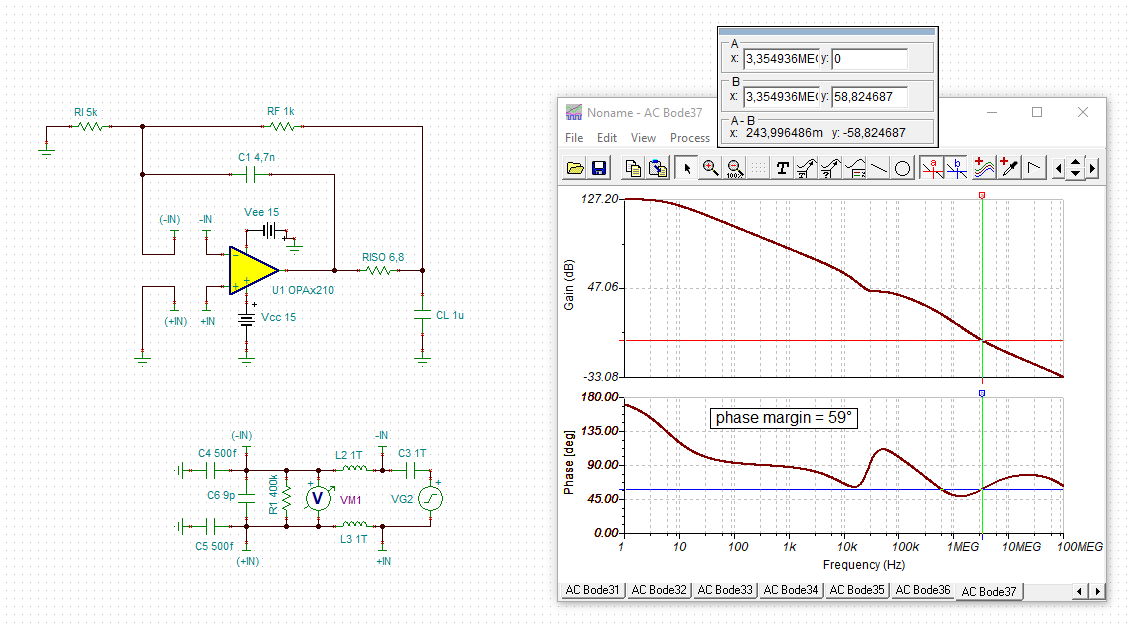tengfei_opa2210.TSC

Kai

• Hi Kai,

I think you are correct. But could you explain how to get C1 4.7n and RISO 6.8 Ohm value? how to calculate C1 4.7n and RISO 6.8 Ohm ?

I always don't know how to get the correct CF and RIOS value.

Tengfei

• Hi Tengfei,

there are formulas and there's even a TI training video on dimensioning this dual feedback compensation circuit in the training video series on stability:

https://training.ti.com/node/1138805

Watch especially the 6. video:

https://training.ti.com/ti-precision-labs-op-amps-stability-isolation-resistor?context=1139747-1139745-14685-1138805-13953

But I like to find a suited circuit by running some simulations.

In your case I have kept RI and RF unchanged because I guess that these two resistors shall be low ohmic because of noise reasons. So I have only played with Riso and C1 (in my circuit). The usual approach is to start with a small Riso like Riso = 1 Ohm e.g. and to step C1 from 10p to about 100n in order to find a phase margin of about 60°. If no such C1 could be found, then increase Riso a bit and try again.

The aim is to get a phase margin of about 60° while Riso and C1 shall be as small as possible. Riso shall be small because the load current causes an unwanted voltag drop across Riso. Also, Riso acts as a low pass filter in combination with CL. And C1 shall be small because it also acts like a low pass filter with the feedback resistors.

Kai

• Hi Kai,

I have watched many times for the videos,  and I think the video solutions are too complicated and I cannot understand.

I only want to get your solutions. You mean you don't  calculate C1  and RISO value, you use different RISO value and different CF to try to get a phase margin 60°?

I

• I think Viode solutions is incorrect. I do a TINA simulation in the attach. f=792k when Aol=20db, then RISO=1/(2*3.14*1uF*792k)=0.2 ohm.

Is this 0.2 Ohm correct?

1447.opa2210_RISO.TSC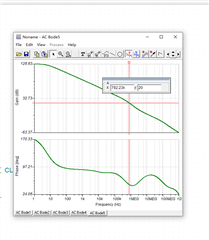• Hi Tengfei,

these are the formulas that are recommended in the 6.video: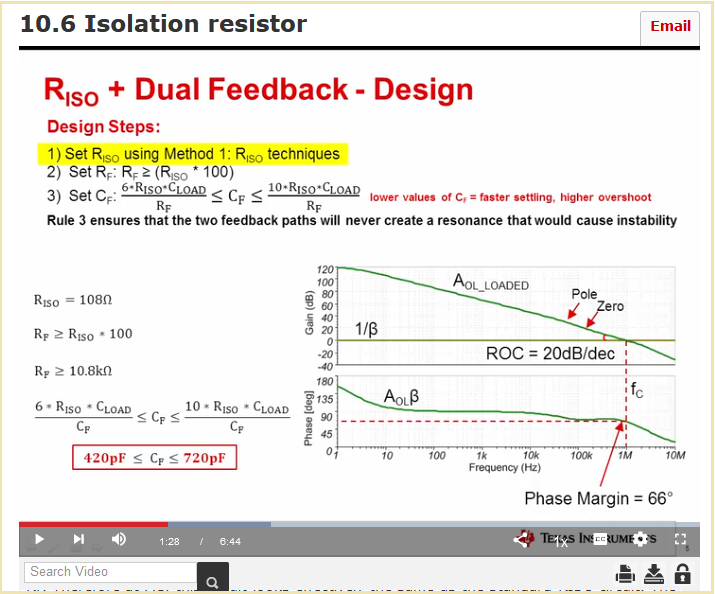Keep in mind that these formulas can only be a starting point because all the many OPAmps arround differ a bit and because the set gain also plays a certain role.

You mean you don't  calculate C1  and RISO value, you use different RISO value and different CF to try to get a phase margin 60°?

From my experience I know from what values to start. Take the above formulas as a starting point, if you want, and do some fine tuning with the simulation.

Kai

• Tengfei,

In order to find the Zo you need to set Riso to zero and determine the second pole frequency - same as 135 degrees phase shift (see below). Once you know the location of the second pole (fp2=52.622kHz), you may back calculate the Zo = 1/(6.28*52,622*1e-6) = ~3ohms.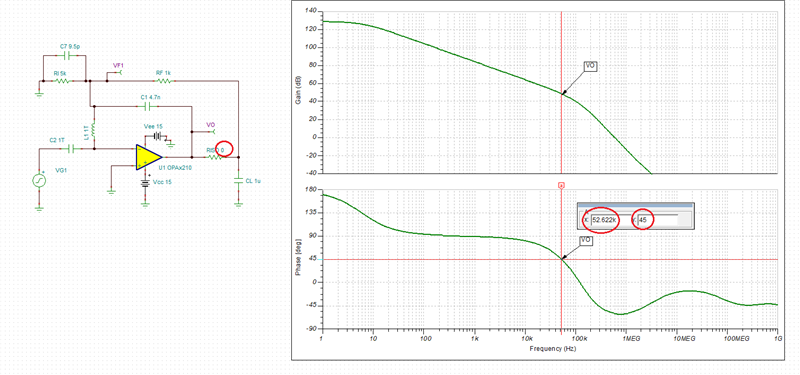You may confirm the value of Zo by looking at the Zo vs frequency graph shown in the OPA210 datasheet - see below.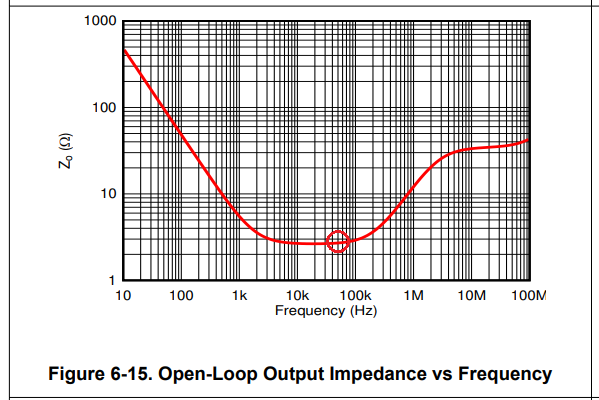Using Riso=Zo of 3ohms assures a minimum required phase margin of 45 degrees (see below) but higher Riso of 6.8ohm that Kai used increases the phase margin to almost 60 degrees, which results in higher stability and thus faster settling time. Since in the application below you employed a dual feedback, you do not need to worry about the increase of the gain error associated with the use of higher Riso as would be the case in a single feedback configuration.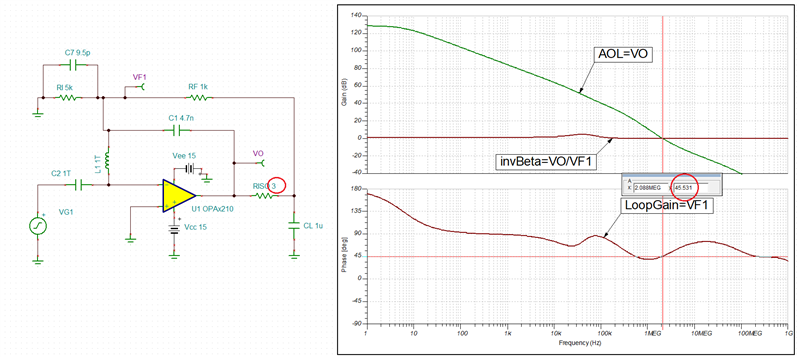• Mark, Kai,

Thanks for your help. I also have some questions as below:

1. According to Video, when AOL=20db, f=268.97k, then RISO=1/(2*3.14*268.97K*1uf）=0.6 ohm, correct?

2. According to Mark solution to calculate RISO, when RISO=0 ohm, I can get the phase margin is 60, f=34.13k. then RISO=1/(2*3.14*34.13k*1uf0=4.7 ohm

Then if RISO=4.7 ohm, the phase margin=52, not 60. why I get this result?

3. To get RISO value, TI Video solution is different Mark's solution. I want to know when I use TI Video solution and when I use Mark's solution?

4. For C1 value, according to TI VIDEO that Kai sent me the formula, if RISO=6.8 ohm, then (  (6*6.8*10^-6)/1000)<C1<(  (10*6.8*10^-6)/1000),

40.8nf<C1<68nf. not 4.7nf.

So my point is TI VIDEO cannot be suitable for my application? How can I calculate C1 value?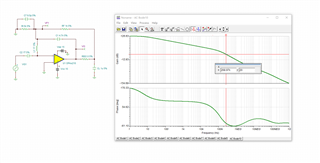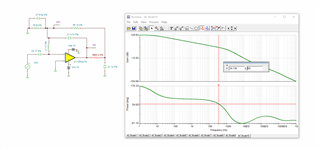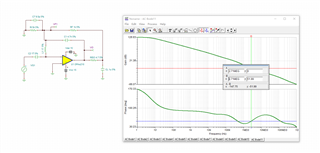• Kai,

Could you share your experience you know about RISO and CF from what value to start?

• Tengfei,

As I wrote in my previous post, first you need to determine the value of internal Ro by finding the frequency of the second pole for a given CL (with Riso=0).

1. Find frequency at the point where phase margin is 45 degrees (90 degree phase shift due to first internal pole plus 45 degree shift at the location of second pole)

2. Since the second pole in op amp transfer function with CL is located at fp2=1/(2*Pi*CL*Ro), you may calculate the value of Ro=1/(2*Pi*CL*fp2)

3. Once you have found Ro, use its value as your starting point for Riso - thus, use Riso = Ro (the circuit should be stable under basically all capacitive loads)

The technique you refer to of using AOL 20dB line to determine value of Riso works only in a simple buffer configuration and NOT in dual feedback amplifier with higher gains.  It attempts to minimize the value of Riso in order to minimize the gain error caused by Riso/RL voltage divider.

Thus, as a general rule, in order to stabilize the circuit, please use the procedure outlined above to determine the starting value of Riso resistor.  Once you determined the value of Riso, for dual feedback solution, use the procedure below: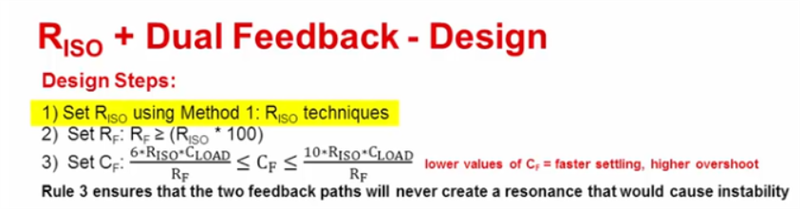• Hi Tengfei,

please relax. You focus way too much on these oversimplified formulas which are only a rough rule of thumb. Use them if you don't have the ability of running a Spice simulation. But when you can run a Spice simulation then find proper values with the simulation.

Also, Riso and CF aren't fixed values. Many other combinations of Riso and CF can also give good results.

I have explained how I do find proper values. I repeat it here:

"The usual approach is to start with a small Riso like Riso = 1 Ohm e.g. and to step CF from 10p to about 100n in order to find a phase margin of about 60°. Step CF by doubling or multiplaying by ten, or use any other step size you want. If no such CF could be found, then increase Riso by doubling and try again.

The aim is to get a phase margin of about 60° while Riso and CF shall be as small as possible. Riso shall be small because the load current causes an unwanted voltag drop across Riso. Also, Riso acts as a low pass filter in combination with CL. And CF shall be small because it also acts like a low pass filter in combination with the feedback resistors."

Kai

• Mark,

1. I have got RISO ways you said.  But for CF, you may check the above information,

if RISO=6.8 ohm, then (  (6*6.8*10^-6)/1000)<C1<(  (10*6.8*10^-6)/1000),

40.8nf<C1<68nf.  4.7nf cannot be included.  Could you explain this?

2. You said" Once you have found Ro, use its value as you starting point for Riso - thus, use Riso = Ro (the circuit should be stable under basically all capacitive loads)", you mean if CL from 1uf increased to be 10uf  or higher 10uf, the circuit should be stable?

• Hi Kai,

if RISO=6.8 ohm, then (  (6*6.8*10^-6)/1000)<C1<(  (10*6.8*10^-6)/1000),

40.8nf<C1<68nf.  4.7nf cannot be included.  Could you explain this?

• Hi Tengfei,

I have already explained why I have chosen a smaller CF.

But, of course, you can also use 47nF instead of 4.7nF:Kai

• 2. You said" Once you have found Ro, use its value as you starting point for Riso - thus, use Riso = Ro (the circuit should be stable under basically all capacitive loads)", you mean if CL from 1uf increased to be 10uf  or higher 10uf, the circuit should be stable?

Yes, with Riso=Ro the circuit will be stable under basically all capacitive loads for as long as the op amp internal third/fourth/etc. pole doesn't come into play - see below.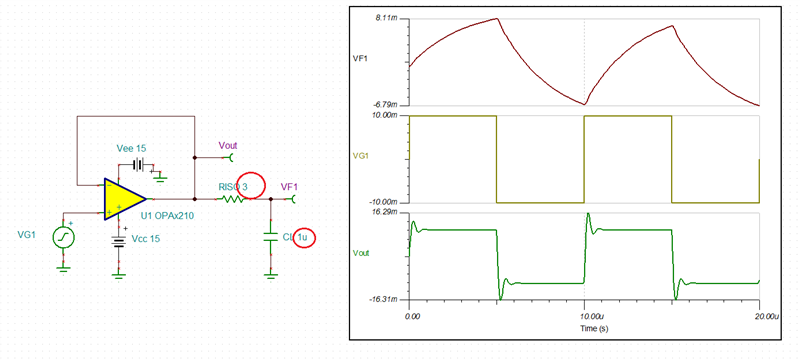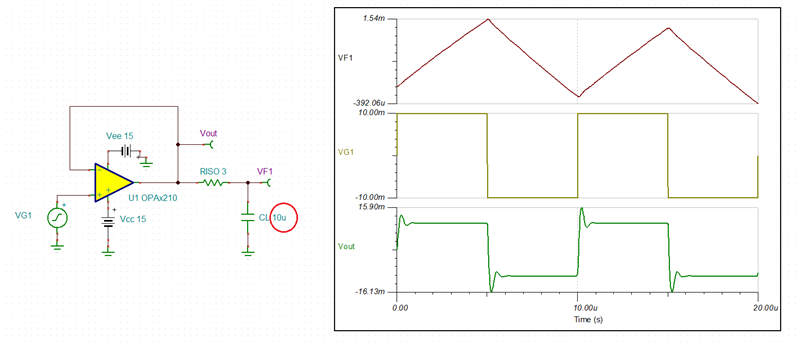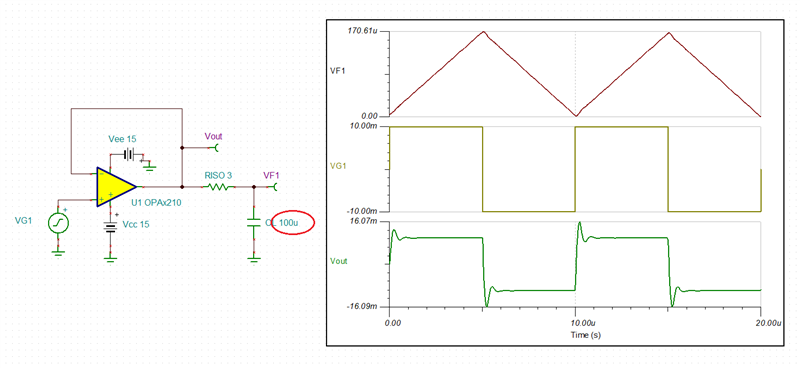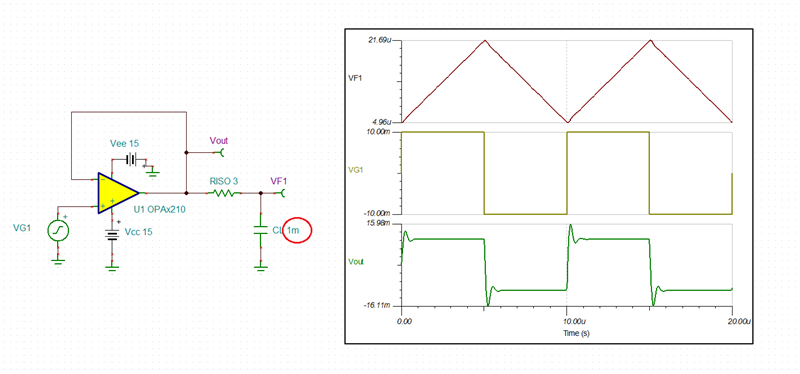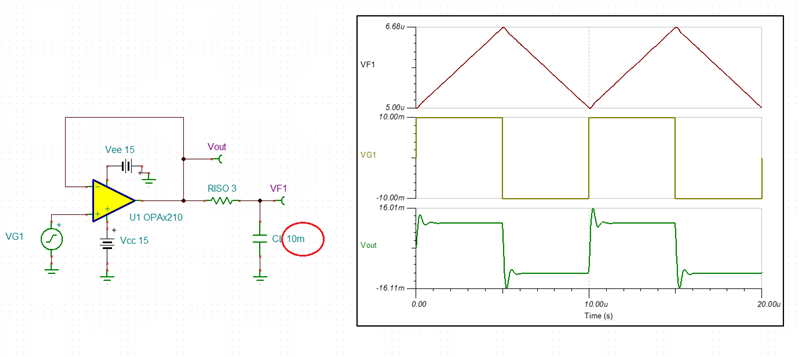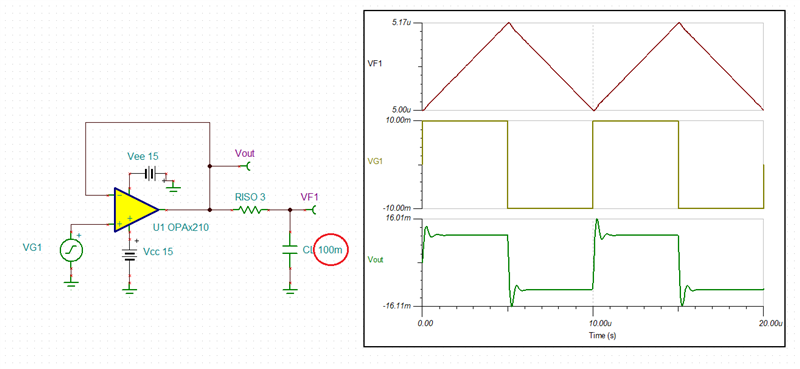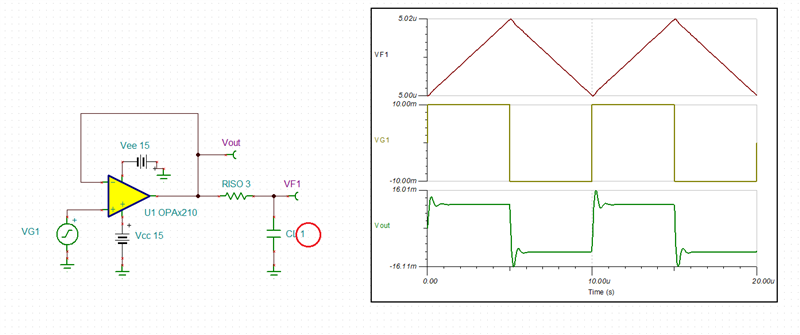Of course, if you use a dual feedback configuration to eliminate the Riso/RL gain error (or any other than buffer configuration), you change the stability of the overall circuit by making it more complex, which may require additional compensation to make it stable - Kai already explained to you one approach how to find the stable solution by trying increasing higher and higher values of R & C components.

• Hi Kai,

Thanks very much! I have got it.

• Good luck